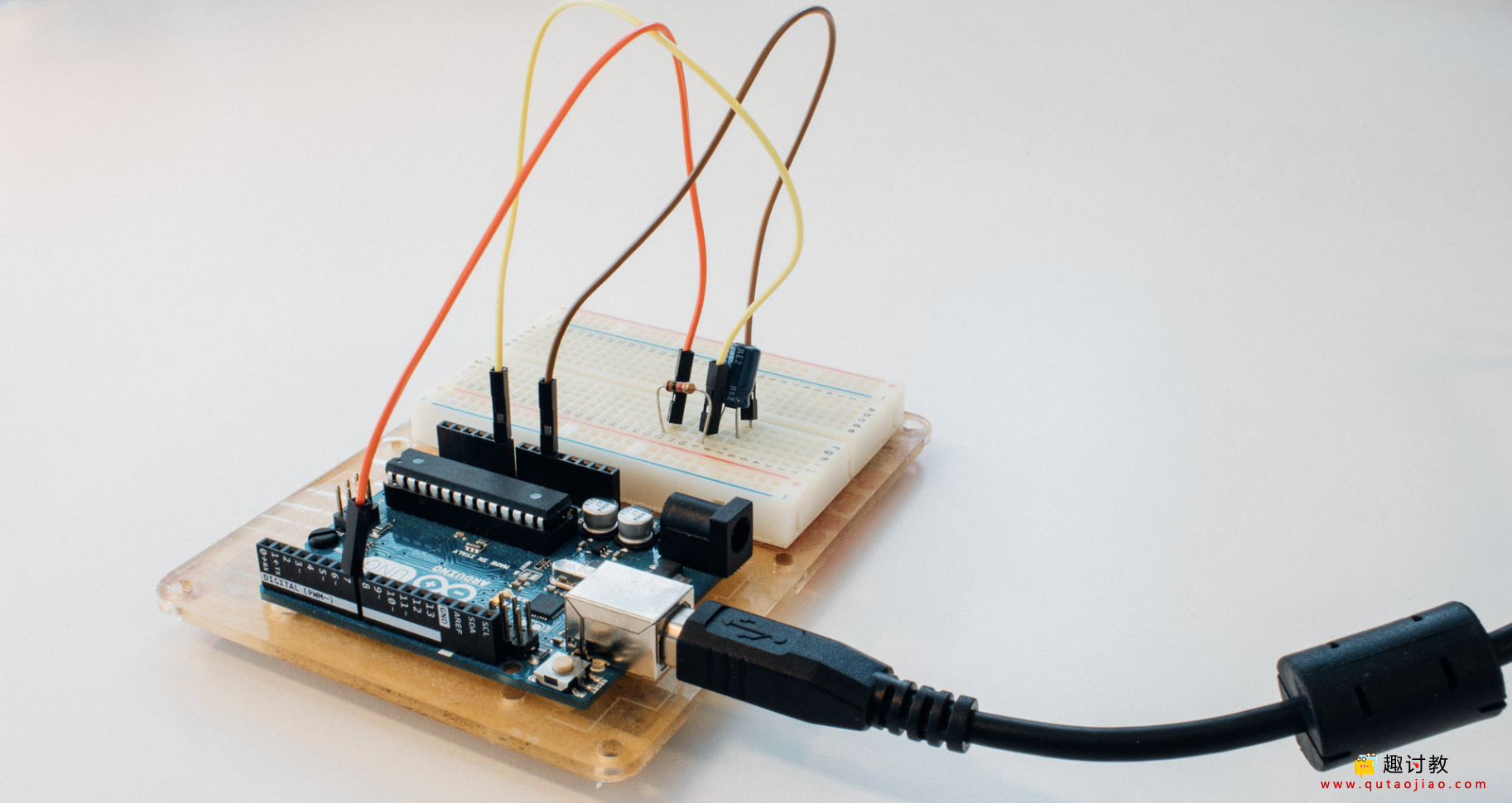# 使用简单的Arduino测量电容值

## 时间常数与电路• 被测电容器与已知电阻（此处为10 k Ohm）串联连接。
• 电容器电路连接到Arduino的引脚7。
• 电容器还连接到Arduino上的模拟输入以测量电压（A0）。## 程序

1. Arduino通过将引脚7设置为低电平来使电容器电路放电。通过读取电容器上的电压来确保电容器为空。
2. 它开始为电容器电路充电并启动计时器。
3. 它持续充电直到电容器达到3,16伏（5 V的〜63,2％）。
4. 当电路达到3.16伏时，它会保存累积的时间值，如果执行的次数少于3次，则会返回到1。
5. 最终答案是所有测量值的平均值，结果将打印在串行端口上！
```#define NO_MEASSUREMENTS 3

#define analog_pin     A0
#define capacitor_pin  7

#define resistor_value   989000.0F // the value on the resistor in Ohms

unsigned long start_time = 0;
unsigned long accumulated_time = 0;

void setup() {
pinMode(capacitor_pin, OUTPUT);

Serial.begin(115200);
}

void loop() {

accumulated_time = 0;

for(uint8_t i = 0; i < NO_MEASSUREMENTS; i++){

// Make sure the capacitor is dicharged
digitalWrite(capacitor_pin, LOW);

// set chargePin HIGH and capacitor charging an
digitalWrite(capacitor_pin, HIGH);
start_time = micros();

// 647 is 63.2% of 1023, which corresponds to full-scale voltage
accumulated_time += (micros() - start_time);
}

float result_time = ((float) accumulated_time) / NO_MEASSUREMENTS;

float micro_F = ( result_time / resistor_value) * 1000;

Serial.print((long)result_time);
Serial.print(" us before tau / ");

print_float(micro_F, 2);
Serial.println(" uF");
}

// from Peter H. Anderson, Baltimore, MD
void print_float(float f, int num_digits)
{
int pows_of_ten = {1, 10, 100, 1000};
int multiplier, whole, fract, d, n;

multiplier = pows_of_ten[num_digits];

if (f < 0.0){
f = -f;
Serial.print("-");
}

whole = (int) f;
fract = (int) (multiplier * (f - (float)whole));

Serial.print(whole);
Serial.print(",");

// print each digit with no leading zero suppression
for (n=num_digits-1; n>=0; n--){
d = fract / pows_of_ten[n];
Serial.print(d);
fract = fract % pows_of_ten[n];
}
}

```

`105404 us before tau / 106,57 uF`

## waiting for host esp8266~启动模式和烧写错误

2019-11-16 15:47:56

## Arduino作为步进电机控制器–通过电位计进行速度和位置控制

2019-11-16 20:37:50

0 条回复 A文章作者 M管理员
暂无讨论，说说你的看法吧

'); })();